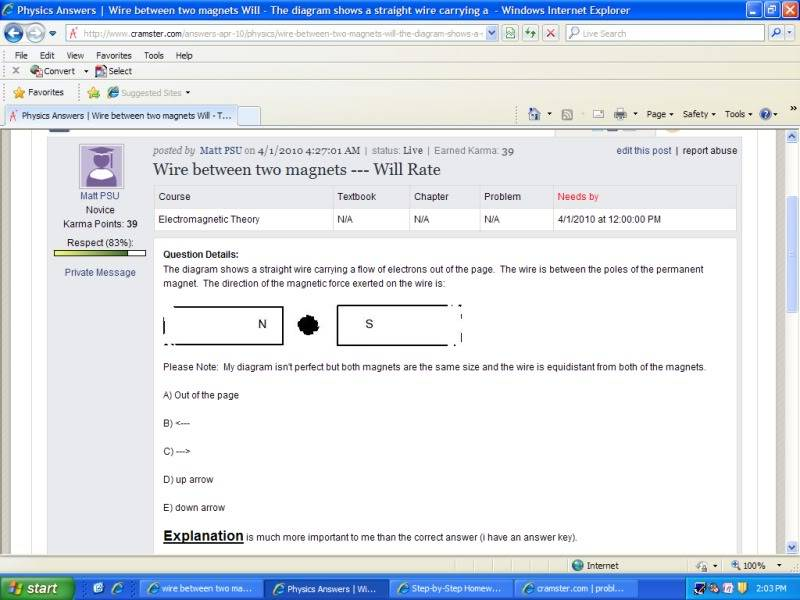# Wire between two magnets

• Matt H

#### Matt H

The diagram shows a straight wire carrying a flow of electrons out of the page. The wire is between the poles of the permanent magnet. The direction of the magnetic force exerted on the wire is:Please Note: My diagram isn't perfect but both magnets are the same size and the wire is equidistant from both of the magnets.

A) Out of the page

B) <---

C) --->

D) up arrow

E) down arrow

An explanation is much more important to me than the correct answer (i have an answer key).

The diagram shows a straight wire carrying a flow of electrons out of the page. The wire is between the poles of the permanent magnet. The direction of the magnetic force exerted on the wire is:

Please Note: My diagram isn't perfect but both magnets are the same size and the wire is equidistant from both of the magnets.

An explanation is much more important to me than the correct answer (i have an answer key).

So how does a wire experience a force? Well, it does it because the current is comprised of electrons (charged particles) moving inside the wire. If those moving charged particles are going through a magnetic field they can experience a force given by the Lorentz equation.

That is F = q V x B.

So let's put it all together. First question. In what direction is the magnetic field? Easy. Magnetic fields are defined to be in the direction from the north pole to the south pole. Hence it is to the right. What direction is the velocity of the charged particles? Well those are given as out of the paper. Thus, what direction is the vector quantity V x B? Well if you take your right hand with thumb up, first finger forward and second finger bent at 90 degrees to the first, the right hand rule is First finger in direction of V, second finger in direction of B and thumb shows direction of result which is up. So it looks like our force is up. But wait! See that q in the equation? That is the charge of the particle. For an electron the value is -e. And that minus sign reverses our result making the force on the wire DOWN. OK? Does that agree with the key?